# python数字图像处理-图像的基本结构和基础知识## 一、python实现BMP图像的读取和显示

BMP文件格式，又称为Bitmap（位图）或是DIB(Device-Independent Device，设备无关位图)，是Windows系统中广泛使用的图像文件格式。由于它可以不作任何变换地保存图像像素域的数据，因此成为我们取得RAW数据的重要来源。Windows的图形用户界面（graphical user interfaces）也在它的内建图像子系统GDI中对BMP格式提供了支持。

BMP文件的数据按照从文件头开始的先后顺序分为四个部分：

2. 位图信息头(bitmap information)：提供图像数据的尺寸、位平面数、压缩方式、颜色索引等信息

3. 调色板(color palette)：可选，如使用索引来表示图像，调色板就是索引与其对应的颜色的映射表

4. 位图数据(bitmap data)：就是图像数据

## 二、python统计图像的直方图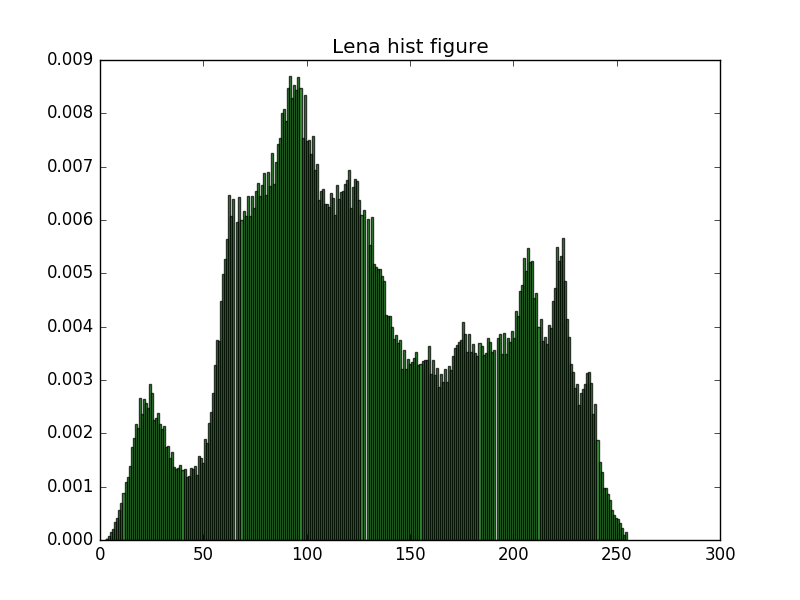## 三、RGB、YIQ、HSI、XYZ颜色空间

### YIQ

YIQ色彩空间通常被北美的电视系统所采用，属于NTSC（National Television Standards Committee）系统。这里Y不是指黄色，而是指颜色的明视度（Luminance），即亮度（Brightness）。其实Y就是图像的灰度值（Gray value），而I和Q则是指色调（Chrominance），即描述图像色彩及饱和度的属性。在YIQ系统中，Y分量代表图像的亮度信息，I、Q两个分量则携带颜色信息，I分量代表从橙色到青色的颜色变化，而Q分量则代表从紫色到黄绿色的颜色变化。

NTSC制为了进一步压缩色度带宽，用色差信号I,Q来代替U,V。若采用U,V色差信号，则色度、亮度信号的共频带部分极大，低端不共频带的亮度信号带宽很小，亮、色干扰大。将彩色图像从RGB转换到YIQ色彩空间，可以把彩色图像中的亮度信息与色度信息分开，分别独立进行处理。

RGB和YIQ的对应关系用下面的方程式表示：

Y=0.299R+0.587G+0.114B
I=0.596R-0.274G-0.322B
Q=0.211R-0.523G+0.312B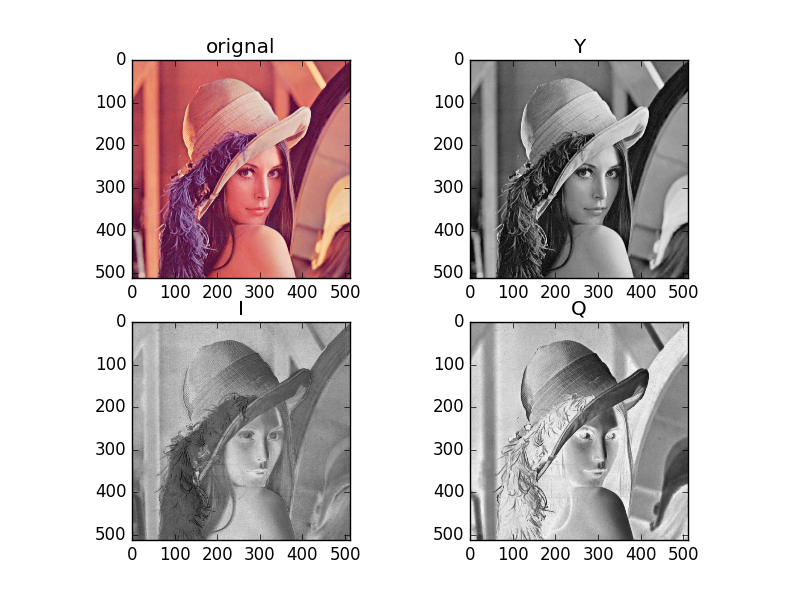### HSI

• 色调H（Hue）：与光波的波长有关，它表示人的感官对不同颜色的感受，如红色、绿色、蓝色等，它也可表示一定范围的颜色，如暖色、冷色等。

• 饱和度S（Saturation）：表示颜色的纯度，纯光谱色是完全饱和的，加入白光会稀释饱和度。饱和度越大，颜色看起来就会越鲜艳，反之亦然。

• 亮度I（Intensity）：对应成像亮度和图像灰度，是颜色的明亮程度。
若将RGB单位立方体沿主对角线进行投影，可得到六边形，这样，原来沿主对角线的灰色都投影到中心白色点，而红色点（1，0，0）则位于右边的角上，绿色点（0，1，0）位于左上角，蓝色点（0，0，1）则位于左下角。

HSI颜色模型的双六棱锥表示，I是强度轴，色调H的角度范围为[0，2π]，其中，纯红色的角度为0，纯绿色的角度为2π/3，纯蓝色的角度为4π/3。饱和度S是颜色空间任一点距I轴的距离。当然，若用圆表示RGB模型的投影，则HSI色度空间为双圆锥3D表示。

HSI模型也可用圆柱来表示，如图9-5所示。若将其展开，并按图9-6进行定义，可得到HSI调色板。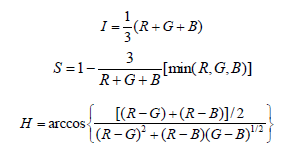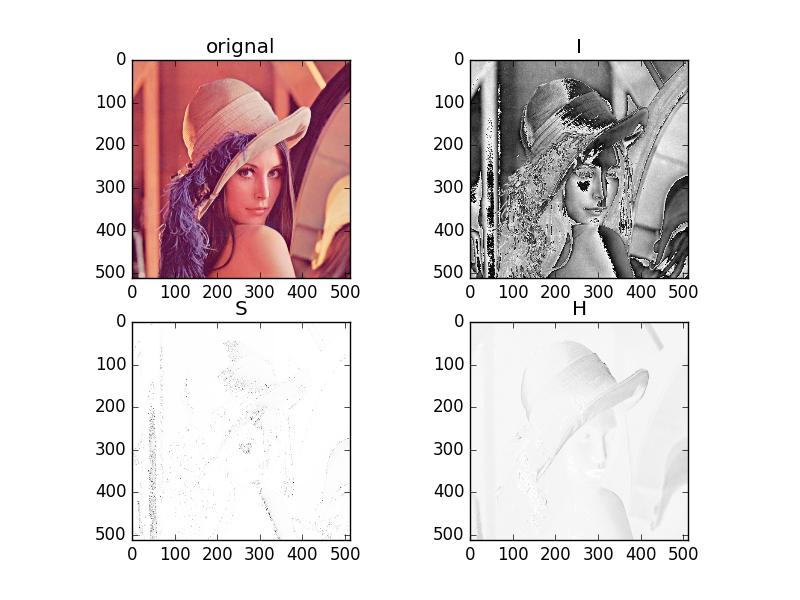### XYZ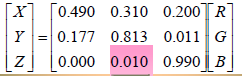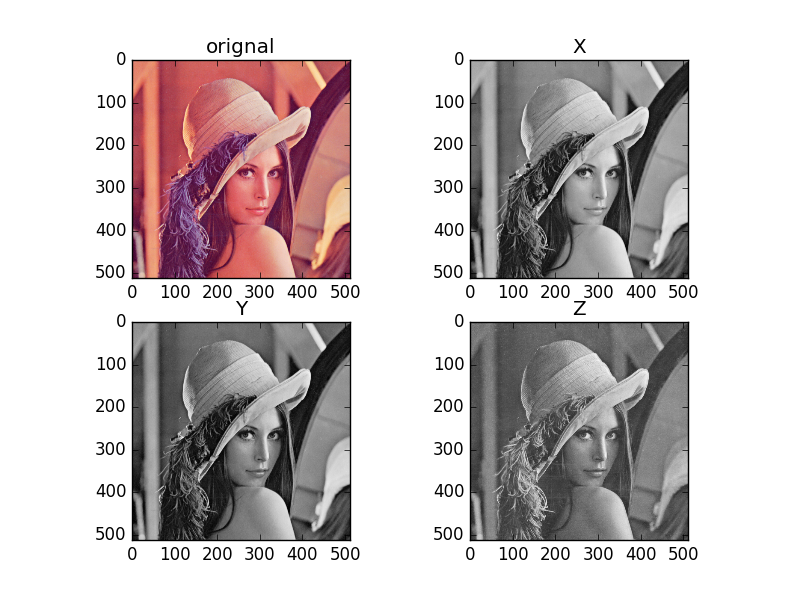## 四、python代码实现

#encoding: utf-8

import numpy as np
from PIL import Image
import matplotlib.pyplot as plt
import math

def show_bmp(img):
# BMP 的读取与显示
plt.figure("LenaRGB.bmp")
plt.title("LenaRGB.bmp")
plt.imshow(img)
plt.show()

def show_hist(img):
# 直方图
plt.figure("Lena hist")
plt.title('Lena hist figure')
arr = img.flatten()
n, bins, patches = plt.hist(arr, bins=256, normed=1, facecolor='green', alpha=0.75)
plt.show()
def show_rgb(img):
# 显示RGB图像
plt.subplot(221)
plt.title('orignal')
plt.imshow(img)
plt.subplot(222)
plt.title('R')
plt.imshow(img[:, :, 0], cmap='gray')
plt.subplot(223)
plt.title('G')
plt.imshow(img[:, :, 1], cmap='gray')
plt.subplot(224)
plt.title('B')
plt.imshow(img[:, :, 2], cmap='gray')
plt.show()

def shou_yiq(img):
# 显示YIQ分量
R = np.mat(img[:, :, 0])
G = np.mat(img[:, :, 1])
B = np.mat(img[:, :, 2])

# aH = np.array([(0.299, 0.587, 0.114),
#               (0.596, -0.274, -0.322),
#               (0.211, -0.523, 0.312)])
Y = 0.299 * R + 0.587 * G + 0.114 * B
I = 0.596 * R - 0.274 * G - 0.322 * B
Q = 0.211 * R - 0.523 * G - 0.312 * B

plt.subplot(221)
plt.title('orignal')
plt.imshow(img)
plt.subplot(222)
plt.title('Y')
plt.imshow(Y, cmap='gray')
plt.subplot(223)
plt.title('I')
plt.imshow(I, cmap='gray')
plt.subplot(224)
plt.title('Q')
plt.imshow(Q, cmap='gray')
plt.show()
# YIQ =

def show_xyz(img):
# 显示YIQ分量
R = np.mat(img[:, :, 0])
G = np.mat(img[:, :, 1])
B = np.mat(img[:, :, 2])

# aH = np.array([(0.299, 0.587, 0.114),
#               (0.596, -0.274, -0.322),
#               (0.211, -0.523, 0.312)])
X = 0.490 * R + 0.310 * G + 0.200 * B
Y = 0.177 * R + 0.813 * G + 0.011 * B
Z = 0.000 * R + 0.010 * G + 0.990 * B

plt.subplot(221)
plt.title('orignal')
plt.imshow(img)
plt.subplot(222)
plt.title('X')
plt.imshow(X, cmap='gray')
plt.subplot(223)
plt.title('Y')
plt.imshow(Y, cmap='gray')
plt.subplot(224)
plt.title('Z')
plt.imshow(Z, cmap='gray')
plt.show()

def show_hsi(img):
rows, cols, dims = img.shape
R = np.mat(img[:, :, 0])
G = np.mat(img[:, :, 1])
B = np.mat(img[:, :, 2])

I = (R + G + B) / 3

E = np.mat(np.ones([rows, cols]))
minRGB = np.mat(img.min(2))
lv = 3 * E / (R + G + B)
S = E - np.multiply(lv, minRGB)

# S = np.mat(np.zeros([rows, cols]))
# for i in range(rows):
#     for j in range(cols):
#         S[i, j] = 1 - 3/(R[i, j] + G[i, j] + B[i, j]) * min([R[i, j], G[i, j], B[i, j]])

H = np.mat(np.zeros([rows, cols]))
for i in range(rows):
for j in range(cols):
up = (R[i, j] - G[i, j] + R[i, j] - B[i, j]) / 2
down = (R[i, j] - G[i, j])**2 + (R[i, j] - B[i, j]) * (G[i, j] - B[i, j])**0.5
H[i, j] = math.acos(up / down)

plt.subplot(221)
plt.title('orignal')
plt.imshow(img)
plt.subplot(222)
plt.title('I')
plt.imshow(I, cmap='gray')
plt.subplot(223)
plt.title('S')
plt.imshow(S, cmap='gray')
plt.subplot(224)
plt.title('H')
plt.imshow(H, cmap='gray')
plt.show()

def main():
img = np.array(Image.open('/home/sun/Desktop/PycharmProjects/Digital_image_process_lxk/lab1/LenaRGB.bmp'))
rows, cols, dims = img.shape
print img.shape
print img.dtype
print img.size
print type(img)

# show_bmp(img)
# show_hist(img)
# show_rgb(img)
# shou_yiq(img)
# show_hsi(img)
# show_xyz(img)

if __name__ == '__main__':
main()

©️2019 CSDN 皮肤主题: 大白 设计师: CSDN官方博客